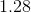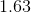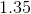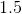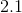SSAT Elementary Level Math : How to find the decimal equivalent of a fraction

Example Questions

Example Question #11 : How To Find The Decimal Equivalent Of A Fraction

Find the decimal equivalent to the fraction. Round to the nearest hundredth.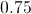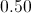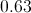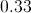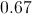Explanation:

If you were to divide a pie into thirds, and then take two of them, you would have more than half of the pie.  Thus you can eliminate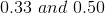.

At this point it's probably best to have memorized the common fractions.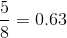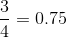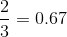Example Question #12 : How To Find The Decimal Equivalent Of A Fraction

Write as an improper fraction in lowest terms: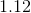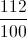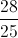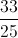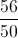Explanation:

The expression is equal to one hundred twelve one-hundredths, so write it as a fraction and reduce as follows: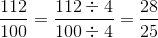Example Question #13 : How To Find The Decimal Equivalent Of A Fraction

Write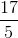as a decimal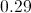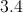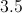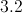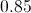Explanation:

Divide 17 by 5: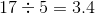Example Question #11 : How To Find The Decimal Equivalent Of A Fraction

What is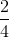equal to in decimal form?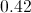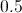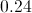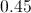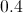Explanation:

To find the decimal equivalent of a fraction, simply divide the top number (numerator) by the bottom number (denominator). In this problem, 2 divided by 4 equals 0.5.

[You can also note that 2 is exactly half of 4, so the fraction will equal .5]

Example Question #11 : How To Find The Decimal Equivalent Of A Fraction

What isequal to in decimal form?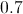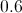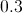Explanation:

To find the decimal equivalent of a fraction, divide the top number (numerator) by the bottom number (denominator). In this problem, 2 divided by 5 equals 0.4!

Example Question #6 : Other Fraction Manipulations

Express the following fraction as a decimal: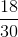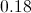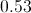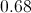Explanation:

To easily express a fraction as a decimal, we need to convert the denominator into a power of. We can do this by dividing both the top and the bottom of the given fraction byto express it in tenths.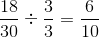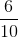, or six tenths, can be expressed as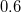.

Example Question #16 : How To Find The Decimal Equivalent Of A Fraction

What isequal to in decimal form?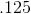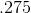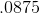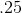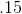Explanation:

To find the decimal equivalent of a fraction, divide the top number (numerator) by the bottom number (denominator). In this problem, 1 divided by 8 equals .125!

Example Question #12 : How To Find The Decimal Equivalent Of A Fraction

What is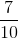equal to in decimal form?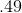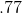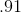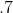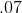Explanation:

To find the decimal equivalent of a fraction, divide the top number (numerator) by the bottom number (denominator). In this problem, 7 divided by 10 equals .7!

Example Question #13 : How To Find The Decimal Equivalent Of A Fraction

What is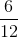equal to in decimal form?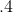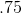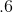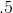Explanation:

To find the decimal equivalent of a fraction, simply divide the top number (numerator) by the bottom number (denominator). In this problem, 6 divided by 12 equals .5!

Example Question #14 : How To Find The Decimal Equivalent Of A Fraction

What is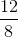equal to in decimal form?